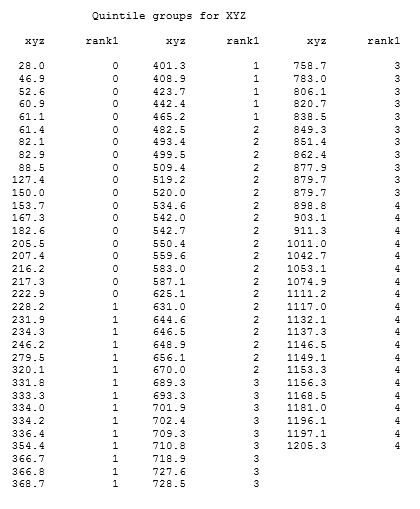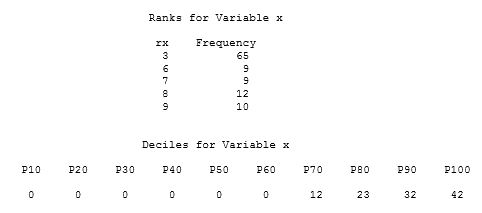SAS users often ask me about the best way to group or bin their data in preparation for additional analysis. Depending on the need, there are several ways to achieve this using SAS procedures or other SAS processing. Creating user-defined formats with PROC FORMAT or using Data step processing are two of the methods that are commonly used.

Often, users need to generate quantile rankings based on the values to create quantile groups like quartiles, quintiles, or deciles. It can be a memory-intensive procedure, but the syntax is pretty simple. For example, you can create quintile groups by specifying GROUPS=5 in the PROC RANK statement. The variable named in the RANKS statement will contain values ranging from 0 to 4 for the groups in the output data set. The following code creates a test data set and shows a simple PROC RANK step. A PROC REPORT step was used to display the results.

```data test; /*  Creating a sample data set  */
do i=1 to 99;
xyz=round((ranuni(i)*1234),.1);
output;
end;
drop i;
run;

proc rank data=test out=out1 groups=5;
var xyz;
ranks rank1;
run;
```The formula PROC RANK uses to create the groups is:

```    group=floor(rank* k/(n+1));

where:
FLOOR is the FLOOR function
rank is the value's order rank. That is the observation number in sort order,
but, for duplicate(tied) values, it's the average observation number.

k is the value of GROUPS=
n is the number of observations having nonmissing values of the ranking variable

```

We can duplicate the results from PROC RANK via a couple of PROC steps and Data step processing using this formula. Using the test data set from above, the following steps create the variables to be used in the formula and applies the formula in a final Data step. The results are the same as those generated by PROC RANK.
```proc sort data=test;
by xyz;
run;

data test2;
set test;
obsnum=_N_;
run;

proc means data=test2 NWAY noprint;
class xyz;
var obsnum;
output out=out2 (drop=_type_ _freq_) mean=order_rank;
run;

proc print data=out2;
run;

data final;
merge test2 out2;
by xyz;
rank2=floor(order_rank *(5/100));
drop obsnum;
/*  floor(rank* k/(n+1));  */
/*  rank=order_rank,  k=5 groups,  n=99 observations */
run;

proc print data=final;
var xyz rank2 order_rank;
run;
```

The GROUPS= option generates quantile ranks. If the data is skewed, that is, if the values of the variable being ranked are not evenly distributed, PROC RANK may not generate the number of groups requested nor groups of equal size. In such cases, there is no option within PROC RANK to ensure that you get the requested number of groups nor groups of equal size.

The following program shows a case when only five groups are generated when GROUPS=10 was requested. You’ll see that the majority of the values are 0. You can look at the decile ranks in the PROC UNIVARIATE output data set to see that the first six deciles generate the same value, 0. Using the formula from above, PROC RANK assigns the 0 values to one group and the other values to 4 other groups.

```data test;  /*  creating sample data set with mostly 0 values */
do i=1 to 40;
x=int(ranuni(i)*44);
output;
end;
do i=41 to 105;
x=0;
output;
end;
run;

proc rank data=test out=test2 groups=10;
var x;
ranks rx;
run;

proc freq data=test2;
tables rx / nopercent nocum;
run;

proc univariate data=test noprint;
var x;
output out=outdec pctlpre=P pctlpts=10 to 100 by 10;
run;

proc print data=outdec;
title 'Deciles for variable x ';
run;
```PROC RANK creates the quantile groups (ranks) in the data set, but users often want to know the range of values in each quantile. There are no options in PROC RANK to determine those ranges. Using other program logic, we can determine those ranges and create a user-defined format containing the ranges.

You can use PROC UNIVARIATE or PROC MEANS to create an output data set containing quantile values for the variable to be ranked. After transposing the output data set from UNIVARIATE or MEANS, DATA step processing is used to create a data set that can be used in the CNTLIN= option of PROC FORMAT. The user-defined format created using PROC FORMAT can then be used in a DATA step or procedure step. An existing Sample showing this method is available at the following link.

Sample 47312: Create a user-defined format containing decile ranges from PROC UNIVARIATE results.

ShareTechnical Support Analyst

Jerry Leonard has worked in Technical Support at SAS for over 18 years. He is currently an Analyst in the SAS Foundations group supporting BASE Procedures and ODS. He has been fortunate to work at SAS for almost 30 years, working previously in support of SAS users and representatives in Latin America. Jerry is an avid runner and enjoys many outdoor activities.

1.How to create a quintile variables of the distribution??

•In a stata

•Rick Wicklin on

Msuva,
You should post programming questions to the SAS Support Communities. In this case, add a BY statement that specifies the strata. For example, if you want quintiles separately for males and females, you can use

``` proc sort data=Have out=Want; by Sex; run;   proc rank data=Want out=RankOut groups=5; by Sex; var MyVar; ranks rank; run;```
2.3.4.